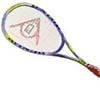#### You may also like### Rain or Shine

Predict future weather using the probability that tomorrow is wet given today is wet and the probability that tomorrow is wet given that today is dry.### Knock-out

Before a knockout tournament with 2^n players I pick two players. What is the probability that they have to play against each other at some point in the tournament?### Squash

If the score is 8-8 do I have more chance of winning if the winner is the first to reach 9 points or the first to reach 10 points?

# How Many Balls?

### Why trythis problem?

To practice interpreting verbal information, writng down expressions for probabilities, putting the information together to obtain an equation and solving the equation.

### Key questions

What are the unknowns?

What are the probabilities?

Can we obtain an equation from the information given?

### Possible support

If some learners can't get started suggest they have a bag and they decide on how many red and how many blue balls are in their bag ( say 4 and 7) and then they work out the probability of drawing two red balls and of drawing two blue balls and of drawing one ball of each colour from their bag. Suggest that after that they use letters for the numbers of red and blue balls (which are unknown) and work out the probabilities in a similar way.

Also try Fixing The Odds

Try Put Out